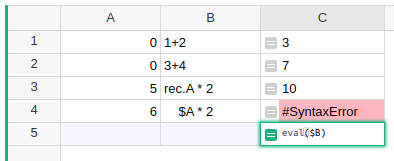How to convert "Text" cell into formula

Example:
Collumn A (text): 1+2
Collumn B (formula): = ???
I want ??? to be 1+2, and in this case 3

=> How to convert text into formula?

1 Like

You can use the Python function eval to evaluate text as Python code:Note that you must use regular Python syntax, i.e. rec.A instead of \$A which is a Grist enhancement. Also eval expects an expression, so multiple statements won’t work.

3 Likes

Thank you, this is what I need
Ps: I hope Grist will create the “grist verson” of function to convert text to formula in the future!